# Texas Go Math Grade 5 Lesson 14.3 Answer Key Graph Number Patterns

Refer to our Texas Go Math Grade 5 Answer Key Pdf to score good marks in the exams. Test yourself by practicing the problems from Texas Go Math Grade 5 Lesson 14.3 Answer Key Graph Number Patterns.

## Texas Go Math Grade 5 Lesson 14.3 Answer Key Graph Number Patterns

Unlock the pattern

When Alice completes each level in her favorite video game, she wins 2 extra lives. Use a graph. How many extra lives will Alice have after 4 levels?
Step 1
Make an input/output table.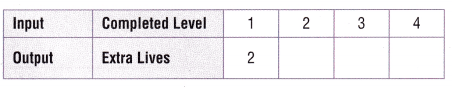Step 2
Write the related pairs of data as ordered pairs.
The input is the x-coordinate and the output is the y-coordinate.
Level 1: (1, 2)
Level 2: _____
Level 3: _____
Level 4: _____
Step 3
Plot the ordered pairs in the coordinate grid.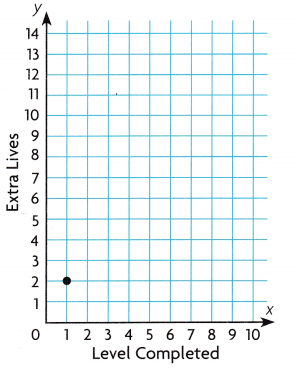So, Alice will have ___ extra lives after completing 4 levels.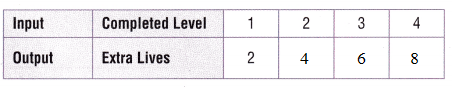Step 2
Write the related pairs of data as ordered pairs.
The input is the x-coordinate and the output is the y-coordinate.
Level 1: (1, 2)
Level 2: (2, 4)
Level 3: (3, 6)
Level 4: (4, 8)
Step 3
Plot the ordered pairs in the coordinate grid.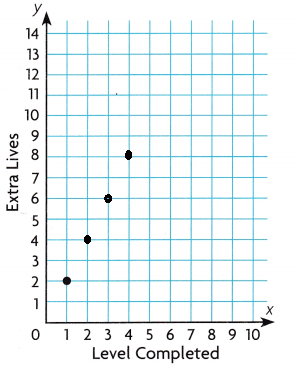So, Alice will have 8 extra lives after completing 4 levels.

Example
The pattern below follows the rule y = x + 3, where y is the number of squares and x is the figure number. Use the pattern to find how many squares will be in Figure 5. Display the pattern in the coordinate grid.Step 1
The pattern in the number of squares is 4, 5, 6, ___, ____.
Step 2
Write ordered pairs using the figure number and the number of squares.
(1, 4)(2, 5)(____, _____)(____, _____)(____, ___)
Step 3
Plot the ordered pairs in the graph.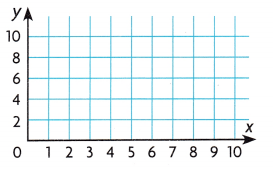Step 1
The pattern in the number of squares is 4, 5, 6, 7, 8
Step 2
Write ordered pairs using the figure number and the number of squares.
(1, 4)(2, 5)(3,6)(4, 7)(5, 8)
Step 3
Plot the ordered pairs in the graph.### Texas Go Math Grade 5 Lesson 14.3 Homework and Practice Answer Key

Use the given rules to complete the pattern. Display the pattern In the coordinate grid.

Question 1.
Multiply the number of nickels by 5 to find the number of pennies that equal the value.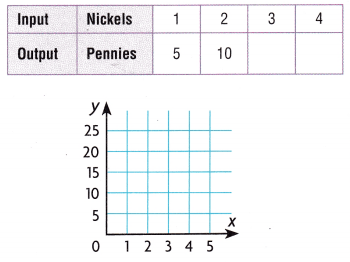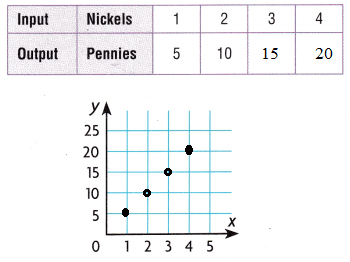Question 2.Problem Solving

Question 3.
H.O.T. Write Math The rule for a pattern is add 4 to the input. Explain how you can use the rule to write ordered pairs that represent the pattern.
I can use an input/output table to find the inputs and outputs. I can use the related input and output numbers to write the ordered pairs.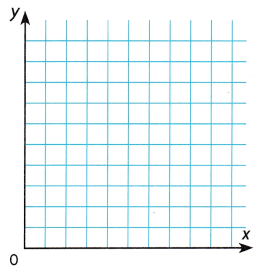Question 4.
Write five ordered pairs that represent the pattern rule multiply input by 3. Display the pattern ¡n the coordinate grid to the right.
(1, 3)(2, 6)(3, 9)(4, 12)(5, 15)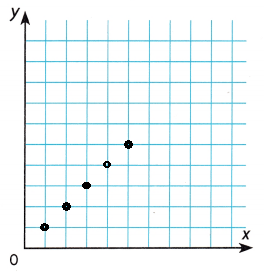Problem Solving

Use the coordinate plane for 5-7.

Question 5.
Multi-Step Emily has a road map with a key that shows an inch on the map equals 5 miles of actual distance. Use the table and the coordinate grid to show the pattern.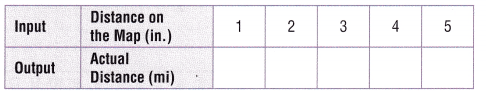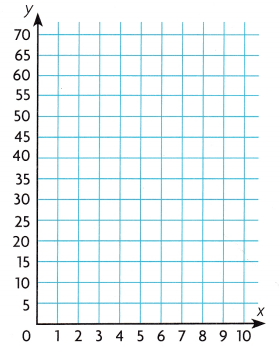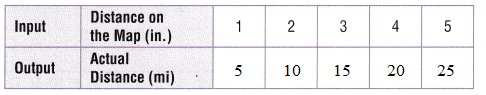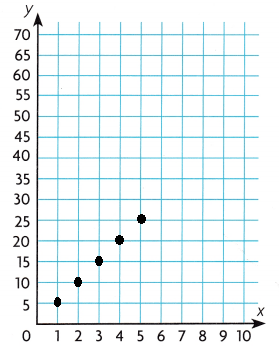Question 6.
Use Graphs What is the actual distance if the distance on the map is 6 inches? Write the ordered pair. Plot a point in the coordinate grid to show the appropriate ordered pair.

Question 7.
H.O.T. What is the distance on the map if the actual distance is 45 miles? Plot a point in the coordinate grid to show the appropriate ordered pair.Question 8.
Ted gets a $10 allowance every week and saves$6 of it. He makes a graph to show his savings, where the x-coordinate represents the number of weeks and they-coordinate represents the number of dollars saved. Which of the following ordered pairs could be on the graph?
(A) (6, 60)
(B) (36, 6)
(C) (1, 60)
(D) (6, 36)

Question 9.
Tina owns a flower shop. The table shows the prices for different numbers of flowers. She makes a graph that compares number of flowers to total price. Which ordered pair should NOT be on the graph?(A) (8, 12)
(B) (6, 4)
(C) (6, 9)
(D) (10, 15)
(B) (6, 4)

Question 10.
Multi-Step Steve mixes 4 cups grape juice with 3 cups ginger ale to make a punch. Steve makes a graph to compare the amounts of juice needed for several batches. Which ordered pairs could be on the graph?
(A) (4, 3), (5, 4), (6, 5)
(B) (3, 4), (5, 6), (6, 7)
(C) (4, 3), (8, 6), (12, 9)
(D) (3, 4), (8, 6), (12, 9)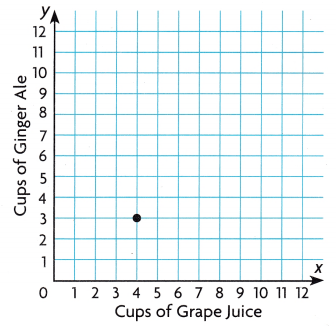(C) (4, 3), (8, 6), (12, 9)

Texas Test Prep

Question 11.
Rob is making a fruit punch for a party. He adds 4 cups of pineapple juice for every cup of orange juice. Which ordered pair shows how many cups of pineapple juice he needs for 3 cups of orange juice?(A) (4, 8)
(B) (1, 2)
(C) (3, 12)
(D) (3, 4)

Use the given rules to complete the pattern. Display the pattern in the coordinate grid.

Question 1.
Multiply the number of cans by 3 to find the number of tennis balls.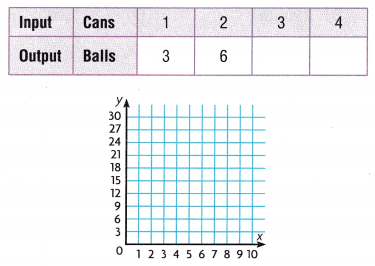Question 2.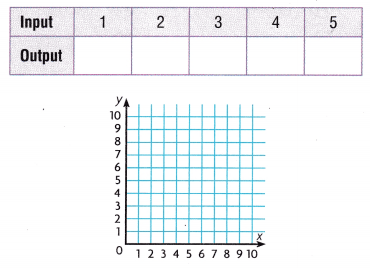Question 3.
Write four ordered pairs that represent the pattern rule multiply input by 4. Display the pattern in the coordinate grid to the right.Question 4.
An architect makes a drawing of a building. One inch in the drawing equals 10 feet in actual measurement. Use the table and the coordinate grid to show the pattern.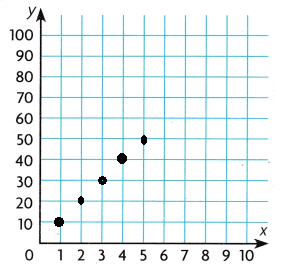Question 5.
What is the actual measurement if the length in the graph drawing is 7 units? Plot a point in the coordinate grid to Show the ordered pair. _____

Lesson Check

Question 6.
Carson purchases tulip bulbs for his garden. Each package contains 6 bulbs. Which ordered pair shows the number of bulbs in 4 packages?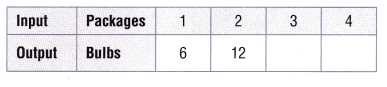(A) (4, 24)
(B) (4, 18)
(C) (3, 16)
(D) (18, 24)

Question 7.
For every $1 the students raise for the new playground equipment fund, the parents association donates$10 to the fund. If the x-coordinate is the amount the students raise and they-coordinate is the amount the parents donate, which ordered pair could be on the graph?
(A) (20, 30)
(B) (20, 10)
(C) (20, 200)
(D) (20, 2,000)

Question 8.
Multi-Step Shakira saves $2 for every$3 she spends. She makes a graph that compares the amount she spends to the amount she saves. Which ordered pairs could be on Shakira’s graph?(A) (6, 9), (8, 12)
(B) (2, 3), (4, 6)
(C) (3, 6), (6, 12)
(D) (9, 6), (12, 8)
Answer: (B) (2, 3), (4, 6)

Question 9.
Multi-Step When the cook at Barney’s Restaurant makes chili, he adds 3 cups of water for every 2 cups of tomato sauce. If you make a graph to compare the amounts of tomato sauce needed for several batches of chili, which ordered pairs could he on the graph?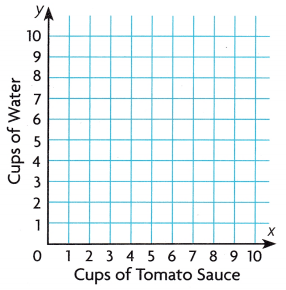(A) (3, 2), (6, 4), (9, 6)
(B) (2, 3), (3, 4), (5,6)
(C) (3, 2), (5, 5), (7, 10)
(D) (2, 3), (4, 6), (6, 9)
Answer: (D) (2, 3), (4, 6), (6, 9)
Thus the answer is option D.

Scroll to Top